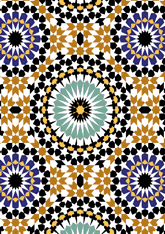data190/MOR1237

## Geometry

• The symmetry group of the tiling is *442 (p4m).
• All the internal angles of the constituent polygons are a multiple of 7.5°.
• Contains two regular two-pointed star polygons with vertex angle of 45°.
• Contains three regular 8-pointed star polygons with vertex angle of 90°.
• Contains one regular 16-pointed star polygon with vertex angle of 45°.
• Contains one regular 24-pointed star polygon with vertex angle of 30°.
• There are 22 non-regular reflective tiles (including 5 kites and one dart) and four reflective pairs.
• The tiling satisfies the two-colour condition.
• The tiling is edge-to-edge.
• As drawn, contains about 1877 polygons.

## References

Publications referenced: